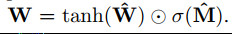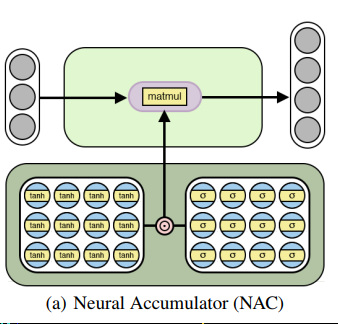medium.com Go back Open original

# Neural Arithmetic and Logic Unit

By anirban ghosh

In recent times the neural network based learning system has got a lot of attention. The reason for this attention is that these systems are getting good at lot many tasks , in some even better than human.This ability for a system to learn things is due to there ability to learn the mappings from the inputs to the outputs. These expert systems do not need to be hard coded with the explicit relations.However , they learn the general properties and features that are good over a wide variety of use cases.
However , it is seen that the neural network donot perform that well once they encounter “numerical values” outside the range they were trained on. Range of Numerical Data refers to the range of the datapoints (all data image , voice , table or language is numerical data for computer) on which a network is trained. In simple language a neural network trained to classify cats from dogs will not be good at classifying a cow. The reason being the network never saw the image of an cow.
This is a drawback of Neural Network that they cannot generalise to data outside the training range of the numerical data that they have encountered.In other words the Neural network cannot extrapolate.
This failure to extrapolate shows that the learnt behaviour of the network is a memorization work instead of general abstraction. Author Andrew Trask and others in their paper Neural Arithmetic Logic Unit have put forward a new architecture that encourage systemic numerical extrapolation.In this architecture , the author proposes addition of a linear activation using simple arithmetic operators like “addition” or “multiplication” etc controlled by learnt gates.In their experiment the authors found that the network got substantially better at generalization both inside (interpolation) and outside (extrapolation) of the range of the numerical values the network was trained on.

As is evident from the image on the left the most non linear functions learn values they are trained on. The error measured by Mean Squared Error (MSE) ramps up as we go outside the training range.

In their training set up the authors used an auto encoder to take a scalar value as input say for example digit 3 , encode the values within its hidden layers , than reconstruct the input values as a linear combination of the last hidden layer to get back the digit 3.

“Autoencoder is a type of artificial neural network used to learn efficient data codings in an unsupervised manner. The aim of an autoencoder is to learn a representation (encoding) for a set of data, typically for the purpose of dimensionality reduction”. Wikipedia.

In their experiment the authors of the paper the authors trained a autoencoder on a number range from -5 to +5 and then tried to reconstruct the numbers from -20 to +20 , these numbers are outside the range of the training data. Most non-linear functions fail to represent number outside the range in which they have seen in their training. “The severity of the failure is directly proportional to the degree of non-linearity in the activation function.”

The Neural Accumulator and Neural Arithmetic Logic Unit.

The neural Accumulator is an special case of linear layer , whose transformation matrix is W ={-1,0,1} , meaning the output is just a addition or subtraction of the input vectors , instead of the non-linear transformation. This makes the output as additions and subtractions of the input rows instead of arbitrary scaling as seen in non-linear functions.But as we know that the backpropagation algorithm can work only in case of continuous values instead of discrete values. So we need to amend the value of W = {-1,0,1} , so that it becomes continuous and differentiable.Here W and M are the weight matrices with kaiming normalization with stable values at {-1,0,1}

The Neural Accumulator (NAC) does the linear transformation of the input vectors as shown in the image below:There is no bais term or non-linearity applied to the output gate.

The NAC is a linear transformation of its inputs. The transformation is a element wise product of its tanh(W) and sigmoid(M).The outputs are linear combinations of the input. A NAC is good at simple arithmetic operations like additions and subtractions. However , if we want to achieve more complex operations like like power or exponentiation then the architecture needs to be changed accordingly to obtain Neural Arithmetic Logic Unit.

Linear Combination in mathematics refers to an expression constructed from a set of terms by multiplying each term by a constant (scalar) and then adding the results. Any expression of the form C =aX+bY where a and b are the scalar constants called the linear weights , is a Linear Combination expression.

The Neural Arithmetic Logic Unit (NALU) is able to learn complex mathematical functions such as multipications and powers. The NALU is an extension on NAC facilitating end-to-end learning.The NALU architecture is shown below :

The NALU has two units NAC units tied together with weights for addition or subtraction (the smaller purple box ) and for multiplication and division (the larger purple box.) controlled by a gate the orange box.

The smaller cell calculates accumulation vector as per the formula: NAC

The larger cell calculates the multiplication and division as it operates in the log space wide this formula :

Altogether these two cell blocks along with the gates allows it to calculate the addition , multiplication , subtraction , division and exponentiation which can extrapolate outside the numbers the networks has seen during training.

We can implement the same in tensorflow as below:

The paper calculated the error using Mean Absolute Error given by the formula: